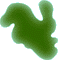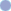Encrypting FilesThis example shows how to encrypt a file.
 SetupFirst we'll set up some convenient variables containing our password, a path to the file we want to encrypt and a path to the location we want the encrypted file saved. ```thePass = "pittabread" theInPath = "c:\myfolder\myfile.txt" theOutPath = "c:\mysecurefolder\myfile.txt"```
 CryptoNext we create an ABCCrypto Crypto object and ask it to encrypt the file using the password we provided as a key. ```Set theCrypto = Server.CreateObject("ABCCrypto2.Crypto") theCrypto.License = "30-day-trial" theCrypto.Password = thePass theCrypto.EncryptFile(theInPath, theOutPath)```